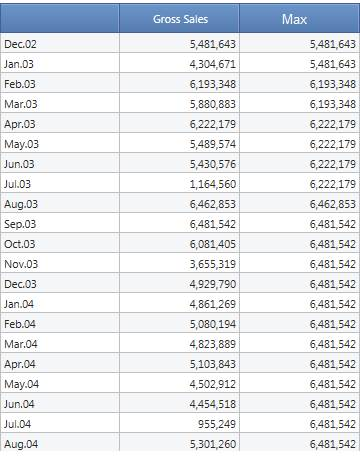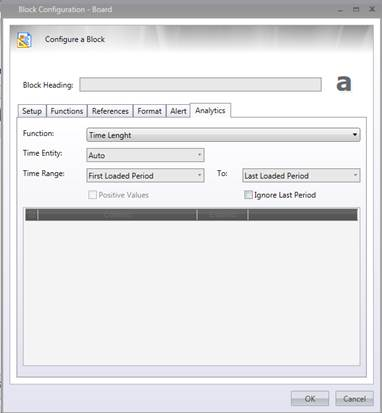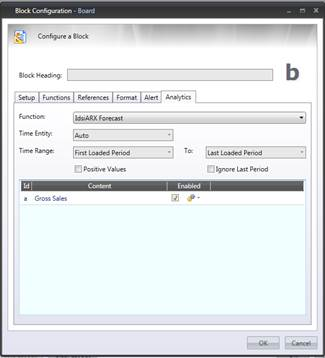﻿ Analytic

# Analytics

## 1Analytical Function

BOARD include a package of analytical functions that easily can be applied directly on the InfoCubes in order to get immediate useful mathematical results without any effort. These functions can be grouped mainly into three different groups: Analytical Functions, Time Series and Statistical Functions; all this functions will make user management easier and faster.

Analytical function work across time entities only, this means that, for example, if I use the maximum function in a dataview, it will return the value of the period with the maximum amount, I cannot use this function to determine the maximum across various products.

The Time functions under the Time Series group will return a series of values in output, so they need by design to be in a layout with at least one time entity by row/column. The other functions return a single value, so they do not need the time entity in the axis. If you use them by any  time entity , the meaning of the function will be “until the period in row/column”.On every row we have the maximum value of the series until that moment,  removing the month from the axis you would just get the overall maximum.

When using those functions in dataflows, of course the row logic is ignored and you will always get the overall maximum.

## 2 Basic Concepts

2.1 Basic Concepts

In order to get in touch with this new feature, you have to:

• open a Data-View’s layout

• double click on the cube type block ( it is not available on algorithms).

• in the Analytics tab select the function from the drop down listthe following settings must be setup:

·           the Function menu allows to select the function from the whole list of available functions

·           time entity drop-down list allows you to decide at which level your period is defined, if I am using maximum, for example, and I choose day as time entity, the function will return the amount of the day that contained the maximum value, otherwise if I select month it will return the value of the best month. You can keep it on “Auto”, in that case it will use the entity on which the cube structure is defined or, if you have the entity in one of the axis, it will take the one by row or column.

·           Time range allows you to take only a part of the time series, deciding which periods the function will consider when calculating the output.

·           The bottom part is only used for the functions IdsiARX.Forecast, Linear Regression and Multiple Linear Regression, it will list all the other cubes in the layout that you might want to use as covariate flagging the covariate checkbox. You can also decide the max lag of the covariate, decide to ignore last period in your forecast and force your series to be always positive. For more details on covariates please check the Predictive Analytics section.List of available analytical functions:

• Analytical Functions:

• Time Length: it returns the amount of periods on which the time series is defined.

• Nascency: it returns the amount of periods passed since the first non-zero value of the series;

• Recency: it returns the amount of periods passed since the last non-zero value of the series;

• Frequency: it returns the amount of non-zero valued  periods in the series;

• Dormancy: it returns the amount of zero valued periods in the series;

• First Value: it returns the first value of the series;

• Last Value: It returns the last value of the series;

• Min Value: it returns the minimum value of the series;

• Min Not Zero Value: it returns the minimum value of the series which is different from 0;

• Max Value: It returns the maximum value of the series;

• Total: it returns the Total value of the series;

• Average: it returns the average value of the series (Total/Time Length);

• Average Not Zero Values: it returns the average of the values that are different from 0 (Total/Frequency);

• Time Series Functions:

• IdsiARX Forecast: given the values in the time series, it returns the forecast according to the IdsiARX algorithm (see the Predictive Analytics section for more details)

• IdsiARX Naive: given the value of the time series it returns the Naive predictor (previous year) for that series.

• Holt Winters: it returns the forecast on future values according to triple exponential smoothing model, the three parameters (alpha, beta, gamma) are configurable.

• Linear Regression: it returns the values of the straight line that best fits the time series in terms of minimum squared error;

• Multiple Linear Regression: same as linear regression but with multiple independent variables.

• Statistical Functions:

• Next Value [IdsiARX Naive]: it returns the next value (first forecasted value) of the series  according to the naive predictor (Previous year);

• Next Value [Linear Regression]: it returns the next value (first forecasted value) of the series according to linear regression model;

• Growth %: it returns the growth of the time series in percentage (this period value - previous period value)*100/ (previous period value);

• Standard deviation: it returns the standard deviation of the series (squared root of the variance)

• Volatility: returns the standard deviation of the return series;

• R Squared: it returns the r squared of the series defined as the ratio between the squared error of the regression model (residual variance) divided by the squared error of the average (total deviance)